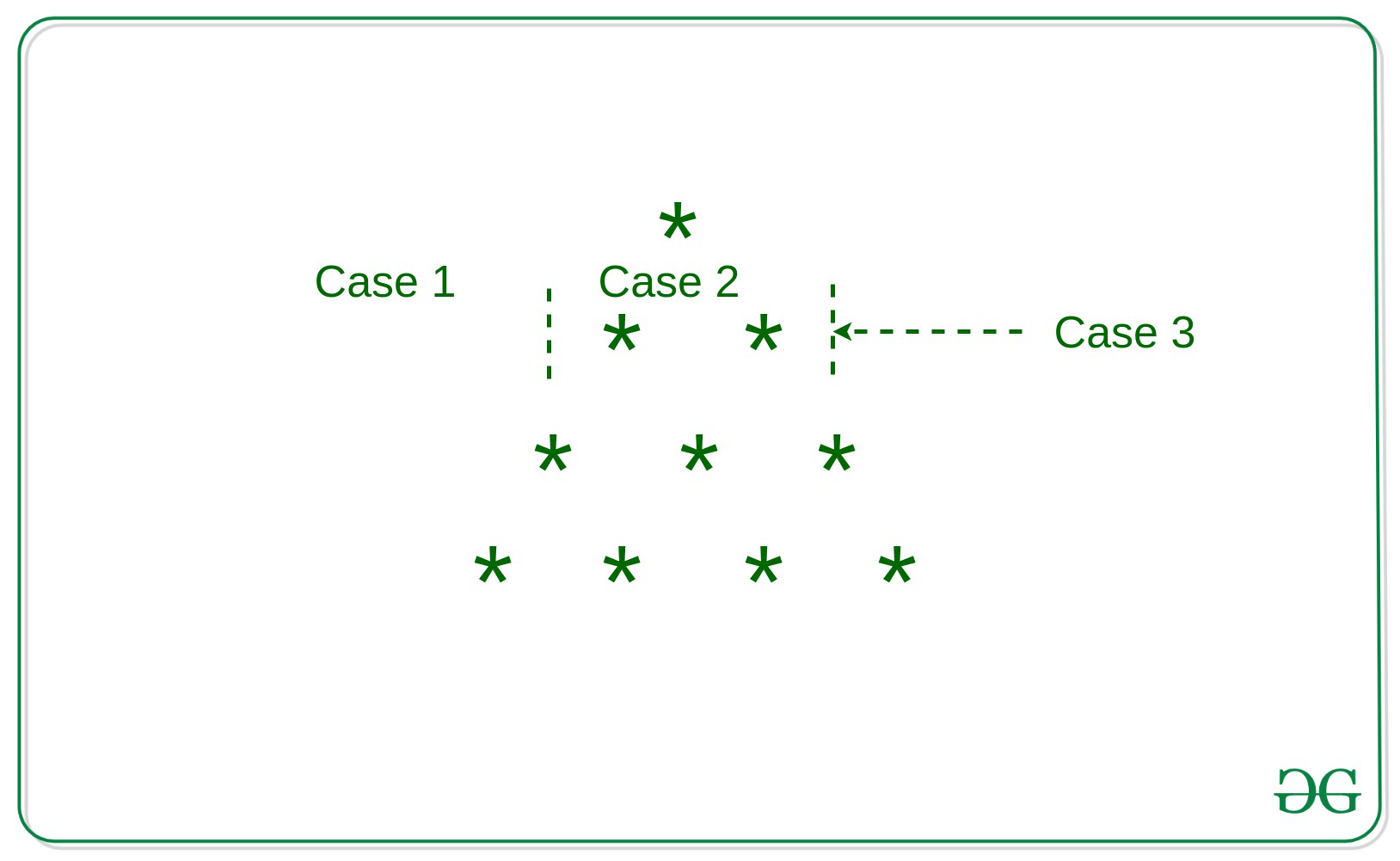GeeksforGeeks App
Open AppBrowser
Continue

# Printing triangle star pattern using a single loop

Given a number N, the task is to print the star pattern in single loop.

Examples:

```Input: N = 9
Output:
*
* *
* * *
* * * *
* * * * *
* * * * * *
* * * * * * *
* * * * * * * *
* * * * * * * * *

Input: N = 5
Output:
*
* *
* * *
* * * *
* * * * * ```

Please Refer article for printing the pattern in two loops as:
Triangle pattern in Java

Approach: The idea is to break a column into three parts and solve each part independently of the others.

• Case 1: Spaces before the first *, which takes care of printing white spaces.
• Case 2: Starting of the first * and the ending of the last * in the row, which takes care of printing alternating white spaces and *.
• Case 3: The ending star essentially tells to print a new line or end the program if we have already finished n rows.
Refer to the image belowBelow is the implementation of the above approach:

## C++

 `// C++ implementation of printing``// star pattern in single loop`` ` `#include ``using` `namespace` `std;`` ` `// Function to print the star``// pattern in single loop``void` `pattern(``int` `n)``{``    ``int` `i, k, flag = 1;``   ` `    ``// Loop to handle number of rows and``    ``// columns in this case``    ``for` `(i = 1, k = 0; i <= 2 * n - 1; i++) {``        ``// Handles case 1``        ``if` `(i < n - k)``            ``cout << ``" "``;`` ` `        ``// Handles case 2``        ``else` `{``            ``if` `(flag)``                ``cout << ``"*"``;``            ``else``                ``cout << ``" "``;`` ` `            ``flag = 1 - flag;``        ``}`` ` `        ``// Condition to check case 3``        ``if` `(i == n + k) {``            ``k++;``            ``cout << endl;`` ` `            ``// Since for nth row we have``            ``// 2 * n- 1 columns``            ``if` `(i == 2 * n - 1)``                ``break``;`` ` `            ``// Reinitializing i as 0,``            ``// for next row``            ``i = 0;``            ``flag = 1;``        ``}``    ``}``}`` ` `// Driver Code``int` `main()``{``    ``int` `n = 6;``   ` `    ``// Function Call``    ``pattern(n);``    ``return` `0;``}`

## Java

 `// Java implementation of printing``// star pattern in single loop``import` `java.util.*;`` ` `class` `GFG{`` ` `// Function to print the star``// pattern in single loop``static` `void` `pattern(``int` `n)``{``    ``int` `i, k, flag = ``1``;``     ` `    ``// Loop to handle number of rows and``    ``// columns in this case``    ``for``(i = ``1``, k = ``0``; i <= ``2` `* n - ``1``; i++) ``    ``{``         ` `        ``// Handles case 1``        ``if` `(i < n - k)``            ``System.out.print(``" "``);`` ` `        ``// Handles case 2``        ``else` `        ``{``            ``if` `(flag == ``1``)``                ``System.out.print(``"*"``);``            ``else``                ``System.out.print(``" "``);`` ` `            ``flag = ``1` `- flag;``        ``}`` ` `        ``// Condition to check case 3``        ``if` `(i == n + k)``        ``{``            ``k++;``            ``System.out.println();``             ` `            ``// Since for nth row we have``            ``// 2 * n- 1 columns``            ``if` `(i == ``2` `* n - ``1``)``                ``break``;`` ` `            ``// Reinitializing i as 0,``            ``// for next row``            ``i = ``0``;``            ``flag = ``1``;``        ``}``    ``}``}`` ` `// Driver code``public` `static` `void` `main(String[] args)``{``    ``int` `n = ``6``;``     ` `    ``// Function Call``    ``pattern(n);``}``}`` ` `// This code is contributed by offbeat`

## Python3

 `# Python3 implementation of ``# printing star pattern in ``# single loop`` ` `# Function to print the star``# pattern in single loop``def` `pattern(n):`` ` `    ``flag ``=` `1`` ` `    ``# Loop to handle number ``    ``# of rows and columns ``    ``# in this case``    ``i ``=` `1``    ``k ``=` `0``    ``while` `i <``=` `2` `*` `n ``-` `1``:``       ` `        ``# Handles case 1``        ``if` `(i < n ``-` `k):``            ``print``(``" "``, end ``=` `"")`` ` `        ``# Handles case 2``        ``else``:``            ``if` `(flag):``                ``print``(``"*"``, end ``=` `"")``            ``else``:``                ``print``(``" "``, end ``=` `"")`` ` `            ``flag ``=` `1` `-` `flag`` ` `        ``# Condition to check case 3``        ``if` `(i ``=``=` `n ``+` `k):``            ``k ``+``=` `1``            ``print``()`` ` `            ``# Since for nth row we ``            ``# have 2 * n- 1 columns``            ``if` `(i ``=``=` `2` `*` `n ``-` `1``):``                ``break`` ` `            ``# Reinitializing i as 0,``            ``# for next row``            ``i ``=` `0``            ``flag ``=` `1`` ` `        ``i ``+``=` `1`` ` `# Driver Code``if` `__name__ ``=``=` `"__main__"``:`` ` `    ``n ``=` `6`` ` `    ``# Function Call``    ``pattern(n)`` ` `# This code is contributed by Chitranayal`

## C#

 `// C# implementation of printing ``// star pattern in single loop ``using` `System; `` ` `class` `GFG{ `` ` `// Function to print the star ``// pattern in single loop ``static` `void` `pattern(``int` `n) ``{ ``    ``int` `i, k, flag = 1; ``     ` `    ``// Loop to handle number of rows and ``    ``// columns in this case ``    ``for``(i = 1, k = 0; i <= 2 * n - 1; i++) ``    ``{ ``         ` `        ``// Handles case 1 ``        ``if` `(i < n - k) ``            ``Console.Write(``" "``); `` ` `        ``// Handles case 2 ``        ``else``        ``{ ``            ``if` `(flag == 1) ``                ``Console.Write(``"*"``); ``            ``else``                ``Console.Write(``" "``); `` ` `            ``flag = 1 - flag; ``        ``} `` ` `        ``// Condition to check case 3 ``        ``if` `(i == n + k) ``        ``{ ``            ``k++; ``            ``Console.WriteLine(); ``             ` `            ``// Since for nth row we have ``            ``// 2 * n- 1 columns ``            ``if` `(i == 2 * n - 1) ``                ``break``; `` ` `            ``// Reinitializing i as 0, ``            ``// for next row ``            ``i = 0; ``            ``flag = 1; ``        ``} ``    ``} ``} `` ` `// Driver code ``public` `static` `void` `Main() ``{ ``    ``int` `n = 6; ``     ` `    ``// Function call ``    ``pattern(n); ``} ``} `` ` `// This code is contributed by sanjoy_62`

## Javascript

 ``

Output

```     *
* *
* * *
* * * *
* * * * *
* * * * * *```

Time complexity: O(n^2) for given n
Auxiliary space: O(1)

My Personal Notes arrow_drop_up